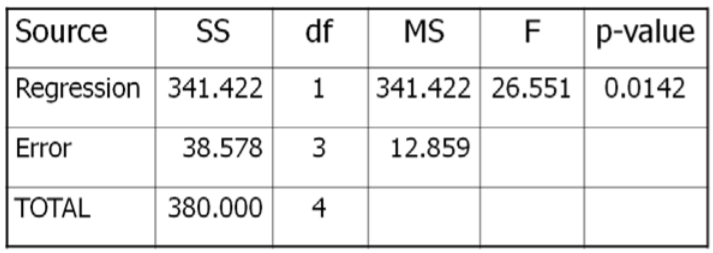# 3.3.4: Hypothesis Test for Simple Linear Regression

$$\newcommand{\vecs}{\overset { \rightharpoonup} {\mathbf{#1}} }$$ $$\newcommand{\vecd}{\overset{-\!-\!\rightharpoonup}{\vphantom{a}\smash {#1}}}$$$$\newcommand{\id}{\mathrm{id}}$$ $$\newcommand{\Span}{\mathrm{span}}$$ $$\newcommand{\kernel}{\mathrm{null}\,}$$ $$\newcommand{\range}{\mathrm{range}\,}$$ $$\newcommand{\RealPart}{\mathrm{Re}}$$ $$\newcommand{\ImaginaryPart}{\mathrm{Im}}$$ $$\newcommand{\Argument}{\mathrm{Arg}}$$ $$\newcommand{\norm}{\| #1 \|}$$ $$\newcommand{\inner}{\langle #1, #2 \rangle}$$ $$\newcommand{\Span}{\mathrm{span}}$$ $$\newcommand{\id}{\mathrm{id}}$$ $$\newcommand{\Span}{\mathrm{span}}$$ $$\newcommand{\kernel}{\mathrm{null}\,}$$ $$\newcommand{\range}{\mathrm{range}\,}$$ $$\newcommand{\RealPart}{\mathrm{Re}}$$ $$\newcommand{\ImaginaryPart}{\mathrm{Im}}$$ $$\newcommand{\Argument}{\mathrm{Arg}}$$ $$\newcommand{\norm}{\| #1 \|}$$ $$\newcommand{\inner}{\langle #1, #2 \rangle}$$ $$\newcommand{\Span}{\mathrm{span}}$$$$\newcommand{\AA}{\unicode[.8,0]{x212B}}$$

We will now describe a hypothesis test to determine if the regression model is meaningful; in other words, does the value of $$X$$ in any way help predict the expected value of $$Y$$?

##### Simple Linear Regression ANOVA Hypothesis Test

Model Assumptions

• The residual errors are random and are normally distributed.
• The standard deviation of the residual error does not depend on $$X$$
• A linear relationship exists between $$X$$ and $$Y$$
• The samples are randomly selected

Test Hypotheses

$$H_o$$:  $$X$$ and $$Y$$ are not correlated

$$H_a$$:  $$X$$ and $$Y$$ are correlated

$$H_o$$:  $$\beta_1$$ (slope) = 0

$$H_a$$:  $$\beta_1$$ (slope) ≠ 0

Test Statistic

$$F=\dfrac{M S_{\text {Regression }}}{M S_{\text {Error }}}$$

$$d f_{\text {num }}=1$$

$$d f_{\text {den }}=n-2$$

Sum of Squares

$$S S_{\text {Total }}=\sum(Y-\bar{Y})^{2}$$

$$S S_{\text {Error }}=\sum(Y-\hat{Y})^{2}$$

$$S S_{\text {Regression }}=S S_{\text {Total }}-S S_{\text {Error }}$$

In simple linear regression, this is equivalent to saying “Are X an Y correlated?”

In reviewing the model, $$Y=\beta_{0}+\beta_{1} X+\varepsilon$$, as long as the slope ($$\beta_{1}$$) has any non‐zero value, $$X$$ will add value in helping predict the expected value of $$Y$$. However, if there is no correlation between X and Y, the value of the slope ($$\beta_{1}$$) will be zero. The model we can use is very similar to One Factor ANOVA.

The Results of the test can be summarized in a special ANOVA table:

Source of Variation Sum of Squares (SS) Degrees of freedom (df) Mean Square (MS) $$F$$
Factor (due to X) $$\mathrm{SS}_{\text {Regression }}$$ 1 $$\mathrm{MS}_{\text {Factor }}=\mathrm{SS}_{\text {Factor }} / 1$$ $$\mathrm{F}=\mathrm{MS}_{\text {Factor }} / \mathrm{MS}_{\text {Error }}$$
Error (Residual) $$\mathrm{SS}_{\text {Error }}$$ $$n-2$$ $$\mathrm{MS}_{\text {Error }}=\mathrm{SS}_{\text {Error }} / \mathrm{n}-2$$
Total $$\mathrm{SS}_{\text {Total }}$$ $$n-1$$
##### Example: Rainfall and sales of sunglasses

Design: Is there a significant correlation between rainfall and sales of sunglasses?

Research Hypotheses:

$$H_o$$:  Sales and Rainfall are not correlated      $$H_o$$:  1 (slope) = 0

$$H_a$$:  Sales and Rainfall are correlated      $$H_a$$:  1 (slope) ≠ 0

Type I error would be to reject the Null Hypothesis and $$t$$ claim that rainfall is correlated with sales of sunglasses, when they are not correlated. The test will be run at a level of significance ($$\alpha$$) of 5%.

The test statistic from the table will be $$\mathrm{F}=\dfrac{\text { MSRegression }}{\text { MSError }}$$. The degrees of freedom for the numerator will be 1, and the degrees of freedom for denominator will be 5‐2=3.

Critical Value for $$F$$ at  $$\alpha$$of 5% with $$df_{num}=1$$ and $$df_{den}=3} is 10.13. Reject \(H_o$$ if $$F >10.13$$. We will also run this test using the $$p$$‐value method with statistical software, such as Minitab.

Data/Results$$F=341.422 / 12.859=26.551$$, which is more than the critical value of 10.13, so Reject $$H_o$$. Also, the $$p$$‐value = 0.0142 < 0.05 which also supports rejecting $$H_o$$.

Conclusion

Sales of Sunglasses and Rainfall are negatively correlated.

3.3.4: Hypothesis Test for Simple Linear Regression is shared under a CC BY-SA license and was authored, remixed, and/or curated by LibreTexts.